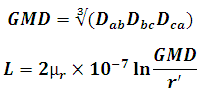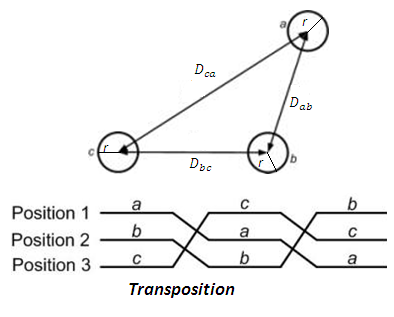# Inductance of Asymmetrical Transposed Three Phase Transmission Line Calculator

This Calctown Calculator calculates the inductance of asymmetrically placed transposed three phase transmission line.

Here µ0 = 4p * 10-7. Please enter the RELATIVE permeability accordingly.

m
m
m
m
unit

#### Result

m
Hwhere

GMD = geometric mean distance

L = Inductance of each individual phase

Dab, Dbc, Dca are respective distances between the lines